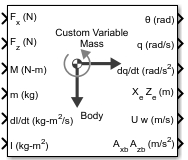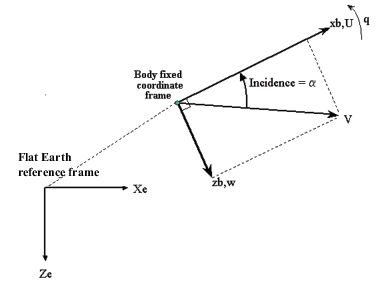Documentation

Custom Variable Mass 3DOF (Body Axes)

Implement three-degrees-of-freedom equations of motion of custom variable mass with respect to body axes

Library

Equations of Motion/3DOFDescription

The Custom Variable Mass 3DOF (Body Axes) block considers the rotation in the vertical plane of a body-fixed coordinate frame about a flat Earth reference frame.The equations of motion are

$\begin{array}{l}{A}_{xb}=\stackrel{˙}{u}=\frac{{F}_{x}}{m}-\frac{\stackrel{˙}{m}Ur{e}_{b}}{m}-qw-g\mathrm{sin}\theta \\ Vr{e}_{b}={\begin{array}{cc}\left[Ure& Wre\right]\end{array}}_{b}\\ {A}_{yb}=\stackrel{˙}{w}=\frac{{F}_{z}}{m}-\frac{\stackrel{˙}{m}Wr{e}_{b}}{m}+qu+g\mathrm{cos}\theta \\ \stackrel{˙}{q}=\frac{M-{\stackrel{˙}{I}}_{yy}q}{{I}_{yy}}\\ \stackrel{˙}{\theta }=q\\ {A}_{be}=\left[\begin{array}{c}{A}_{xe}\\ {A}_{ze}\end{array}\right]=\frac{{F}_{b}-\stackrel{˙}{m}Ur{e}_{b}}{m}-\overline{g}\\ {A}_{bb}=\left[\begin{array}{c}{A}_{xb}\\ {A}_{yb}\end{array}\right]\end{array}$

where the applied forces are assumed to act at the center of gravity of the body. Ureb and Wreb are the relative velocities of the mass flow ($\stackrel{˙}{m}$) being added to or ejected from the body in body-fixed axes.

Parameters

Main

Units

Specifies the input and output units:

Units

Forces

Moment

Acceleration

Velocity

Position

Mass

Inertia

Metric (MKS)

Newton

Newton meter

Meters per second squared

Meters per second

Meters

Kilogram

Kilogram meter squared

English (Velocity in ft/s)

Pound

Foot pound

Feet per second squared

Feet per second

Feet

Slug

Slug foot squared

English (Velocity in kts)

Pound

Foot pound

Feet per second squared

Knots

Feet

Slug

Slug foot squared

Mass Type

Select the type of mass to use:

 Fixed Mass is constant throughout the simulation. Simple Variable Mass and inertia vary linearly as a function of mass rate. Custom Variable Mass and inertia variations are customizable.

The Custom Variable selection conforms to the previously described equations of motion.

Initial velocity

A scalar value for the initial velocity of the body, (V0).

Initial body attitude

A scalar value for the initial pitch attitude of the body, (θ0).

Initial incidence

A scalar value for the initial angle between the velocity vector and the body, (α0).

Initial body rotation rate

A scalar value for the initial body rotation rate, (q0).

Initial position (x,z)

A two-element vector containing the initial location of the body in the flat Earth reference frame.

Gravity Source

Specify source of gravity:

 External Variable gravity input to block Internal Constant gravity specified in Acceleration due to gravity
Acceleration due to gravity

A scalar value for the acceleration due to gravity used if internal gravity source is selected. If gravity is to be neglected in the simulation, this value can be set to 0. This parameter appears if you set Gravity source to Internal.

Include mass flow relative velocity

Select this check box to add a mass flow relative velocity port. This is the relative velocity at which the mass is accreted or ablated.

Include inertial acceleration

Select this check box to enable an additional output port for the accelerations in body-fixed axes with respect to the inertial frame. You typically connect this signal to the accelerometer.

State Attributes

Assign unique name to each state. You can use state names instead of block paths during linearization.

• To assign a name to a single state, enter a unique name between quotes, for example, 'velocity'.

• To assign names to multiple states, enter a comma-delimited list surrounded by braces, for example, {'a', 'b', 'c'}. Each name must be unique.

• If a parameter is empty (' '), no name assignment occurs.

• The state names apply only to the selected block with the name parameter.

• The number of states must divide evenly among the number of state names.

• You can specify fewer names than states, but you cannot specify more names than states.

For example, you can specify two names in a system with four states. The first name applies to the first two states and the second name to the last two states.

• To assign state names with a variable in the MATLAB® workspace, enter the variable without quotes. A variable can be a character vector, cell array, or structure.

Velocity: e.g., {'u', 'w'}

Specify velocity state names.

Default value is ''.

Pitch attitude: e.g., 'theta'

Specify pitch attitude state name.

Default value is ''.

Position: e.g., {'Xe', 'Ze'}

Specify position state names.

Default value is ''.

Pitch angular rate: e.g., 'q'

Specify pitch angular rate state name.

Default value is ''.

Inputs and Outputs

InputDimension TypeDescription
FirstScalarContains the force acting along the body x-axis, (Fx).
Second ScalarContains the force acting along the body z-axis, (Fz).
ThirdScalarContains the applied pitch moment, (M).
Fourth (Optional)VectorContains the rate of change of mass, ($\stackrel{˙}{m}$) (positive if accreted, negative if ablated).
FifthScalarContains the mass, (m).
SixthScalarContains the rate of change of inertia tensor matrix, $\left({\stackrel{˙}{I}}_{yy}\right)$.
SeventhScalarContains the inertia tensor matrix, (Iyy).
Eighth (Optional)Scalar Contains the gravity in the selected units.

Ninth (Optional)

Two-element vectorContains one or more relative velocities at which the mass is accreted to or ablated from the body in body axes.
OutputDimension TypeDescription
FirstScalarContains the pitch attitude, within ±pi, in radians (θ).
SecondScalarContains the pitch angular rate, in radians per second (q).
ThirdScalarContains the pitch angular acceleration, in radians per second squared $\left(\stackrel{˙}{q}\right)$.
FourthTwo-element vectorContains the location of the body, in the flat Earth reference frame, (Xe, Ze).
FifthTwo-element vectorContains the velocity of the body resolved into the body-fixed coordinate frame, (u, w).
SixthTwo-element vectorContains the acceleration of the body resolved into the body-fixed coordinate frame, (Ax, Az).
Seventh (Optional)Two-element vectorContains the accelerations in body-fixed axes with respect to inertial frame (flat Earth). You typically connect this signal to the accelerometer.

Introduced in R2006a

Aerospace Blockset Documentation평가판 신청